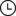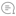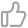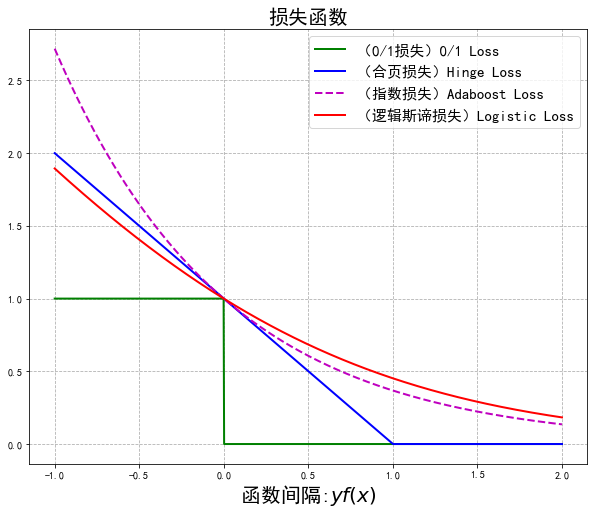# 【机器学习基础】常见二分类损失函数、距离度量的Python实现机器学习初学者 |314天前000

import numpy as np

## 1.欧氏距离(Euclidean distance)

def euclidean(x, y):    return np.sqrt(np.sum((x - y)**2))

## 2.曼哈顿距离(Manhattan distance)

def manhattan(x, y):    return np.sum(np.abs(x - y))

## 3.切比雪夫距离(Chebyshev distance)

def chebyshev(x, y):    return np.max(np.abs(x - y))

## 4.闵可夫斯基距离(Minkowski distance)

def minkowski(x, y, p):    return np.sum(np.abs(x - y) ** p) ** (1 / p)

## 5.汉明距离(Hamming distance)

def hamming(x, y):    return np.sum(x != y) / len(x)6.二分类损失函数在二分类的监督学习中，支持向量机、逻辑斯谛回归与最大熵模型、提升方法各自使用合页损失函数、逻辑斯谛损失函数、指数损失函数，分别写为：这 3 种损失函数都是 0-1 损失函数的上界，具有相似的形状。(见下图，由代码生成）import numpy as npimport mathimport matplotlib.pyplot as pltplt.rcParams['font.sans-serif'] = ['SimHei']plt.rcParams['axes.unicode_minus'] = Falseplt.figure(figsize=(10,8))x = np.linspace(start=-1, stop=2, num=1001, dtype=np.float)logi = np.log(1 + np.exp(-x)) / math.log(2)boost = np.exp(-x)y_01 = x < 0y_hinge = 1.0 - xy_hinge[y_hinge < 0] = 0plt.plot(x, y_01, 'g-', mec='k', label='（0/1损失）0/1 Loss', lw=2)plt.plot(x, y_hinge, 'b-', mec='k', label='（合页损失）Hinge Loss', lw=2)plt.plot(x, boost, 'm--', mec='k', label='（指数损失）Adaboost Loss', lw=2)plt.plot(x, logi, 'r-', mec='k', label='（逻辑斯谛损失）Logistic Loss', lw=2)plt.grid(True, ls='--')plt.legend(loc='upper right',fontsize=15)plt.xlabel('函数间隔:$yf(x)$',fontsize=20)plt.title('损失函数',fontsize=20)plt.show()总结本文用Python实现了常见的几种距离度量、损失函数，欢迎收藏！往期精彩回顾适合初学者入门人工智能的路线及资料下载机器学习在线手册深度学习在线手册AI基础下载（pdf更新到25集）本站qq群1003271085，加入微信群请回复“加群”获取一折本站知识星球优惠券，复制链接直接打开：https://t.zsxq.com/yFQV7am喜欢文章，点个在看00

暂无评论~~
Ctrl+Enter